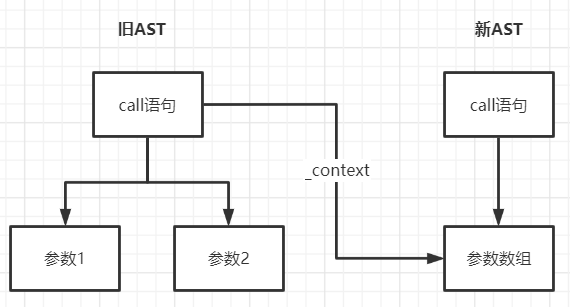• 编译原理
• 发布于 2个月前
• 182 热度
0 评论

`let name = "touryung";`

```const WHITESPACE = /\s/; // 空格
const NUMBERS = /[0-9]/; // 数字
const LETTERS = /[a-z]/i; // 变量

function tokenizer(input) {
let current = 0; // 当前识别到的下标
let tokens = []; // token 数组

while (current < input.length) {
// 遍历
let char = input[current]; // 当前遍历到的字符

// 不同的 token 识别操作 www.duidaima.com

throw new TypeError(`I dont know what this character is: \${char}`);
}

}```

```// 识别左括号
if (char === "(") {
tokens.push({ type: "paren", value: "(" }); // 压入描述当前 token 的对象
current++;
continue;
}
// 识别右括号
if (char === ")") {
tokens.push({ type: "paren", value: ")" });
current++;
continue;
}```

```// 空格，不处理
if (WHITESPACE.test(char)) {
current++;
continue;
}```

```// 数字，循环获取数值
if (NUMBERS.test(char)) {
let value = ""; // 匹配的数字赋初值

while (NUMBERS.test(char)) { // 遍历，如果还能匹配就累加
value += char;
char = input[++current];
}

tokens.push({ type: "number", value }); // 压入描述当前 token 的对象
continue;
}

// 变量，和 number 类似
if (LETTERS.test(char)) {
let value = "";

while (LETTERS.test(char)) {
value += char;
char = input[++current];
}

tokens.push({ type: "name", value });
continue;
}

// 字符串，前后的 "" 需要跳过
if (char === '"') {
let value = "";
char = input[++current]; // 跳过前面的引号

while (char !== '"') { // 结束条件，匹配到末尾的引号
value += char;
char = input[++current];
}

char = input[++current]; // 跳过后面的引号
tokens.push({ type: "string", value });
continue;
}```

1.在字符串识别时需要跳过前后的引号，只匹配中间具体的值；
2.在中间进行遍历的时候结束条件是匹配到末尾的引号。

```let source = "(add 2 (subtract 4 2))"; // 源代码
let tokens = tokenizer(source);
console.log(tokens);```

```[
{ "type": "paren", "value": "(" },
{ "type": "name", "value": "add" },
{ "type": "number", "value": "2" },
{ "type": "paren", "value": "(" },
{ "type": "name", "value": "subtract" },
{ "type": "number", "value": "4" },
{ "type": "number", "value": "2" },
{ "type": "paren", "value": ")" },
{ "type": "paren", "value": ")" }
]```

```function parser(tokens) {
let current = 0;

function walk() {
let token = tokens[current];

// 将不同的 token 转化为 AST 节点

throw new TypeError(token.type);
}

let ast = {
// 此为 AST 树最外层结构，是固定的
type: "Program",
body: [],
};

while (current < tokens.length) {
// 遍历 token 数组，构建树结构
ast.body.push(walk());
}

return ast;
}```

```// 构建整数节点 www.duidaima.com
if (token.type === "number") {
current++;
return {
type: "NumberLiteral",
value: token.value,
};
}
// 构建字符串节点
if (token.type === "string") {
current++;
return {
type: "StringLiteral",
value: token.value,
};
}```

```if (token.type === "paren" && token.value === "(") {
// 左括号开始
token = tokens[++current]; // 跳过左括号
let node = {
// 函数调用节点
type: "CallExpression",
name: token.value,
params: [],
};
token = tokens[++current]; // 跳过 name

// 只要不是右括号，就递归收集参数节点
while (!(token.type === "paren" && token.value === ")")) {
node.params.push(walk()); // 添加参数
token = tokens[current];
}

current++; // 跳过右括号
return node;
}```

```if (value === "(") {
// ...
while (!value === ")") {
// ...
}
}```

```{
"type": "Program",
"body": [
{
"type": "CallExpression",
"params": [
{
"type": "NumberLiteral",
"value": "2"
},
{
"type": "CallExpression",
"name": "subtract",
"params": [
{
"type": "NumberLiteral",
"value": "4"
},
{
"type": "NumberLiteral",
"value": "2"
}
]
}
]
}
]
}```

```// 访问（参数）数组
function traverseArray(array, parent) {
array.forEach((child) => traverseNode(child, parent));
}```

```function traverseNode(node, parent) {
let method = visitor[node.type]; // 去除当前类型的方法

if (method && method.enter) {
// 执行对应 enter 方法
method.enter(node, parent);
}

switch (
node.type // 对不同类型节点执行不同的遍历操作
) {
case "Program":
traverseArray(node.body, node);
break;
case "CallExpression":
traverseArray(node.params, node);
break;
case "NumberLiteral":
case "StringLiteral":
break;
default:
throw new TypeError(node.type);
}
}```

1.在旧的 AST 树中加入一个 _context 上下文属性，指向新的 AST 树的数组节点
2.当遍历旧 AST 数组节点的子元素时，将转化后的子元素放入它的父元素的 _context 属性中
3.根据 JavaScript 引用类型的特点，此时就实现了将转化和的节点放入新 AST 树的目的。```function transformer(ast) {
let newAst = {
// 新 AST 树的最外层结构
type: "Program",
body: [],
};

// _context 用于遍历旧子节点时压入新 ast
ast._context = newAst.body;

let visitor = {
// 不同类型的节点访问（转化）方法
};

traverser(ast, visitor); // 开始遍历旧 AST 树
return newAst;
}```

```{
NumberLiteral: {
enter(node, parent) {
parent._context.push({ // 压入新 AST 树
type: "NumberLiteral",
value: node.value,
});
},
},
StringLiteral: {
enter(node, parent) {
parent._context.push({
type: "StringLiteral",
value: node.value,
});
},
}
}```

```{
CallExpression: {
enter(node, parent) {
let expression = { // 新 AST 树的函数调用节点
type: "CallExpression",
callee: {
type: "Identifier",
name: node.name,
},
arguments: [],
};

node._context = expression.arguments; // 参数数组处理

// 如果当前的函数调用不是嵌套在别的函数调用中
if (parent.type !== "CallExpression") {
expression = {
type: "ExpressionStatement",
expression: expression,
};
}

parent._context.push(expression);
},
},
}```

```{
"type": "Program",
"body": [
{
"type": "ExpressionStatement",
"expression": {
"type": "CallExpression",
"callee": {
"type": "Identifier",
},
"arguments": [
{
"type": "NumberLiteral",
"value": "2"
},
{
"type": "CallExpression",
"callee": {
"type": "Identifier",
"name": "subtract"
},
"arguments": [
{
"type": "NumberLiteral",
"value": "4"
},
{
"type": "NumberLiteral",
"value": "2"
}
]
}
]
}
}
]
}```

```function codeGenerator(node) {
switch (node.type) {
case "Program":
return node.body.map(codeGenerator).join("\n");
case "ExpressionStatement":
return `\${codeGenerator(node.expression)};`;
case "CallExpression": // 生成函数调用式
return `\${codeGenerator(node.callee)}(\${node.arguments
.map(codeGenerator)
.join(", ")})`;
case "Identifier": // 生成变量名
return node.name;
case "NumberLiteral":
return node.value; // 生成数字
case "StringLiteral":
return `"\${node.value}"`; // 生成字符串（别忘了两边的引号）
default:
throw new TypeError(node.type);
}
}```